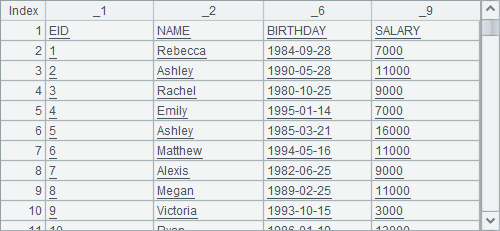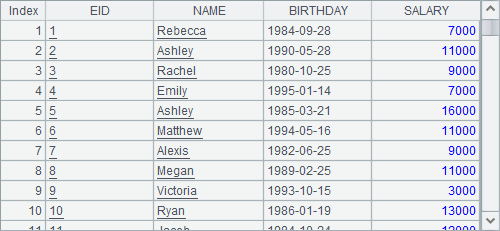# 自定义函数

## 调用自定义函数的基本方法

package test;

public class Calc01 {

public static Double distance1(Number loc) {

double len = Math.abs(loc.doubleValue());

len = Math.round(len*1000)/1000d;

return Double.valueOf(len);

}

}

 A 1 -12.34567 2 =invoke(test.Calc01.distance1,A1) 3 =invoke(test.Calc01.distance1, -512)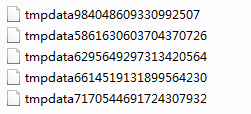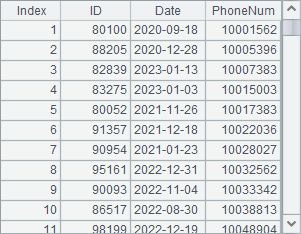public static Double distance2(Number loc1, Number loc2) {

double len = Math.abs(loc1.doubleValue()-loc2.doubleValue());

len = Math.round(len*1000)/1000d;

return Double.valueOf(len);

}

 A 1 =invoke(test.Calc01.distance1,-12.3456) 2 =invoke(test.Calc01.distance2,12,-12)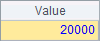## 根据需要返回结果

public static void distance3(Number loc1, Number loc2) {

double len = Math.abs(loc1.doubleValue()-loc2.doubleValue());

len = Math.round(len*1000)/1000d;

System.out.println(Double.toString(len));

}

 A 1 >invoke(test.Calc01.distance3,-12.3,15)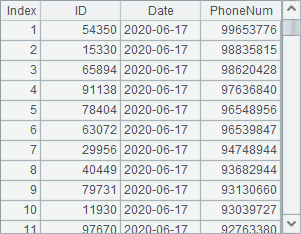整数 java.lang.Integer 长整数 java.lang.Long 浮点数 java.lang.Double 长实数 java.math.BigInteger 实数 java.lang.Number 布尔型 java.lang.Boolean 字符串 java.lang.String 日期 java.sql.Date 时间 java.sql.Time 日期时间 java.sql.TimeStamp 序列 com.raqsoft.dm.Sequence 序表 com.raqsoft.dm.Table 二进制数据 byte[]

public static String distance4(Number loc1, Number loc2) {

double len = Math.abs(loc1.doubleValue()-loc2.doubleValue());

len = Math.round(len*1000)/1000d;

return Double.toString(len);

}

public static Sequence distance5(Number loc1, Number loc2) {

double len = Math.abs(loc1.doubleValue()-loc2.doubleValue());

len = Math.round(len*1000)/1000d;

com.raqsoft.dm.Sequence result = new com.raqsoft.dm.Sequence();

return result;

}

 A 1 =invoke(test.Calc01.distance2,-12.3,15) 2 =invoke(test.Calc01.distance4,-12.3,15) 3 =invoke(test.Calc01.distance5,-12.3,15)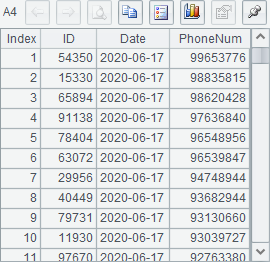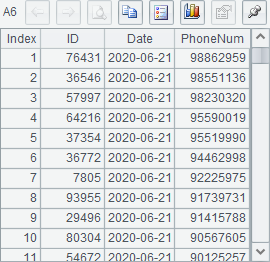## 使用序列类型的参数

public static Double distance6(com.raqsoft.dm.Sequence seq1, com.raqsoft.dm.Sequence seq2) {

int len1 = seq1.length();

int len2 = seq2.length();

double x1 = len1 > 0 ? ((Number) seq1.get(1)).doubleValue(): 0;

double x2 = len2 > 0 ? ((Number) seq2.get(1)).doubleValue(): 0;

double y1 = len1 > 1 ? ((Number) seq1.get(2)).doubleValue(): 0;

double y2 = len2 > 1 ? ((Number) seq2.get(2)).doubleValue(): 0;

double len = Math.sqrt((x1-x2)*(x1-x2)+(y1-y2)*(y1-y2));

len = Math.round(len*1000)/1000d;

return Double.valueOf(len);

}

 A 1 [1,1] 2 [3,3] 3 =invoke(test.Calc01.distance6,A1,A2)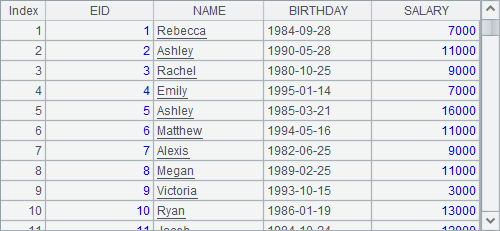## 使用自定义游标

package test;

public class RandDataCursor implements com.raqsoft.dm.ILineInput {

private int rowno = 0;

private int range = 1000;

public RandDataCursor(Integer rg) {

this.range = rg;

}

public Object[] readLine() throws java.io.IOException {

rowno++;

Object[] result = new Object;

result = Integer.valueOf(rowno);

result = Integer.valueOf((int) (Math.random() * range ));

return result;

}

public boolean skipLine() throws java.io.IOException {

rowno++;

if (rowno <= 10000) return true;

return false;

}

public void close() throws IOException {

rowno = 10001;

}

}

package test;

public class Calc02 {

public static test.RandDataCursor getCursor(Integer range) {

RandDataCursor rdc = new RandDataCursor(range);

return rdc;

}

}

 A 1 =invoke(test.Calc02.getCursor,1000000) 2 =invoke(com.raqsoft.dm.UserUtils.newCursor,A1,"") 3 >A2.skip(100) 4 =A2.fetch@x(100)

A1中，返回自定义游标类，RandDataCursorA2中，调用UserUtils中的newCursor方法，将A1中的结果作为参数传入，A2中的数据即为集算器中的游标数据了。A3中跳过前100条记录后，A4中取出100条数据后关闭游标，A4中结果如下：